## Filters

Sort by :
Clear All
Q

Q. 1.    Find the area of following parallelograms:

(ii)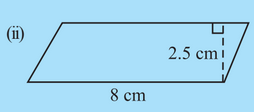Area of the parallelogram is the product of base and height

Find the area of following parallelograms:

(i)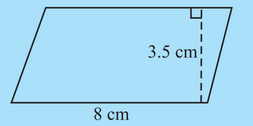Area of the parallelogram is the product of base and height

In Fig 11.31,

(b) Which is larger, perimeter of smaller square or the circumference of the circle?The arc length of the circle is slightly greater than the side length of the inner square. Therefore circumference of the inner circle is greater than the inner square

In Fig

(a) Which square has a larger perimeter?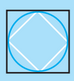The outer square has a larger perimeter. Since each side of the inner square forms a triangle. The length of the third side of a triangle is less than the sum of the other two lengths.

Q 4    Here is an incomplete net for making a cube. Complete it in at least two different
ways. Remember that a cube has six faces. How many are there in the net here?
(Give two separate diagrams. If you like, you may use a squared sheet for easy
manipulation.)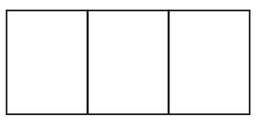There are three faces in the given net. The two possibilities of forming nets are

Q 2     Dice are cubes with dots on each face. Opposite faces of a die always have a total of seven dots on them. Here are two nets to make dice (cubes); the numbers inserted in each square indicate
the number of dots in that box.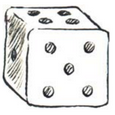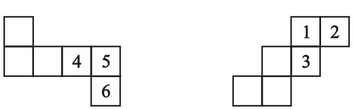Insert suitable numbers in the blanks, remembering that the number on the
opposite faces should total to 7.

The sum of the opposite sides of a dice =7

Convert the following:

(iv)

The conversion is done as follows

Convert the following:

(iii)

1m = 100cm Therefor

Convert the following:

(ii)

ha represents hectare 1 ha is 10000 m2 therefor

Convert the following:

(i)

1 cm = 10 mm therefor

Find the area of the following parallelograms:

(iii)  In a parallelogram , and the perpendicular from  on is

Area of parallelogram = the product of base and height

Q. 1.    Each of the following rectangles of length 6 cm and breadth 4 cm is composed of congruent polygons. Find the area of each             polygon.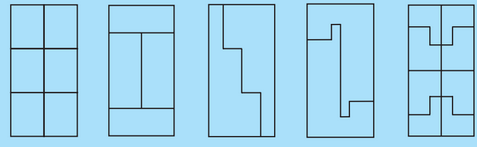The total area of rectangle  i) The first rectangle is divided into 6 equal parts. So the area of each part will be one-sixth of the total area  ii) The rectangle is divided into 4 equal parts, area of each part= one-forth area of rectangle= 6 cm2 iii) and (iv) are divided into two equal parts. Area of each part will be one half the total area of rectangle= 12  cm2 v) Area of rectangle is...

Give two examples where the area does not increase when perimeter increases.

Area of a rectangle with length = 20cm, breadth = 5 cm is 100cm2 and perimeter is 50 cm. A rectangle with sides 50 cm and 2 cm ha area = 100cm2  but perimeter is 104 cm

Give two examples where the area increases as the perimeter increases.

A square of side 1m has perimeter 4 m and area 1 m2. When all sides are increased by 1m then perimeter =8m and area= 4 m2. Similarly if we increase the length from 6m to 9m and breadth from 3m to 6m  of a rectangular the perimeter and area will increase

What would you need to find, area or perimeter, to answer the following?

How much plastic sheet do you need to cover a rectangular swimming pool?

plastic sheet need to cover is the area of the rectangular swimming pool

What would you need to find, area or perimeter, to answer the following?

What distance would you cover by taking two rounds of a triangular park?

Distance covered by taking round a triangular park is equal to the circumference of the triangular park

What would you need to find, area or perimeter, to answer the following?

Q. 2.     What is the length of a wire required to fence a rectangular flower bed?

The length of the wire to fence a flower bed is the circumference of the flower bed

What would you need to find, area or perimeter, to answer the following?

How much space does a blackboard occupy?

The space of the board includes whole area of the board